Graph all the things

analyzing all the things you forgot to wonder about

# A Tournament

2015-04-24

A tournament is a set of nodes in which each pair of nodeshas exactly one edge between them: eitheror. In tournament terms, either playerbeatsorbeats. Let's make a tournament where each playerpicks an angle, wherebeatsifis counterclockwise from: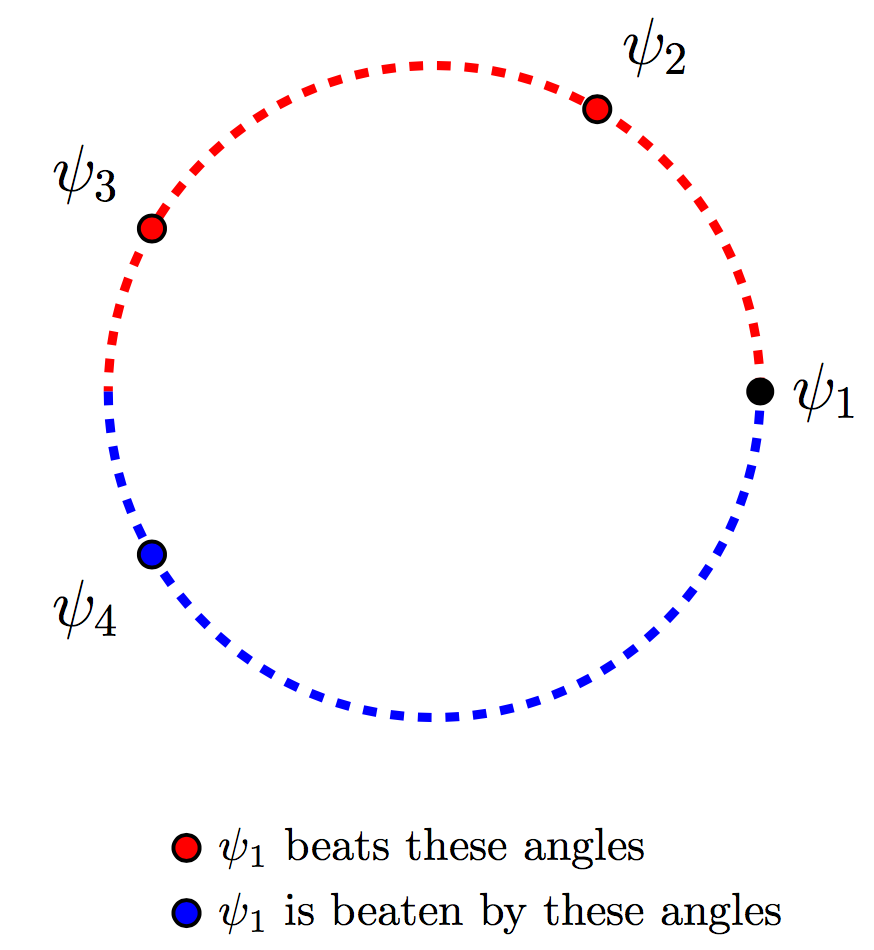Ifbeats, we say. This tournament has some neat properties:

• If there is any cycle, then it has a subcycleof length 3.
• The tournament has a cycle if and only if each angle beats at least one other angle. From another perspective, there is a cycle as long as the angles do not all lie on one half of the circle.
Using these and some cool facts from my previous post, we can find a set of 3 points on a sphere to use for interpolation at another given point. What the hell?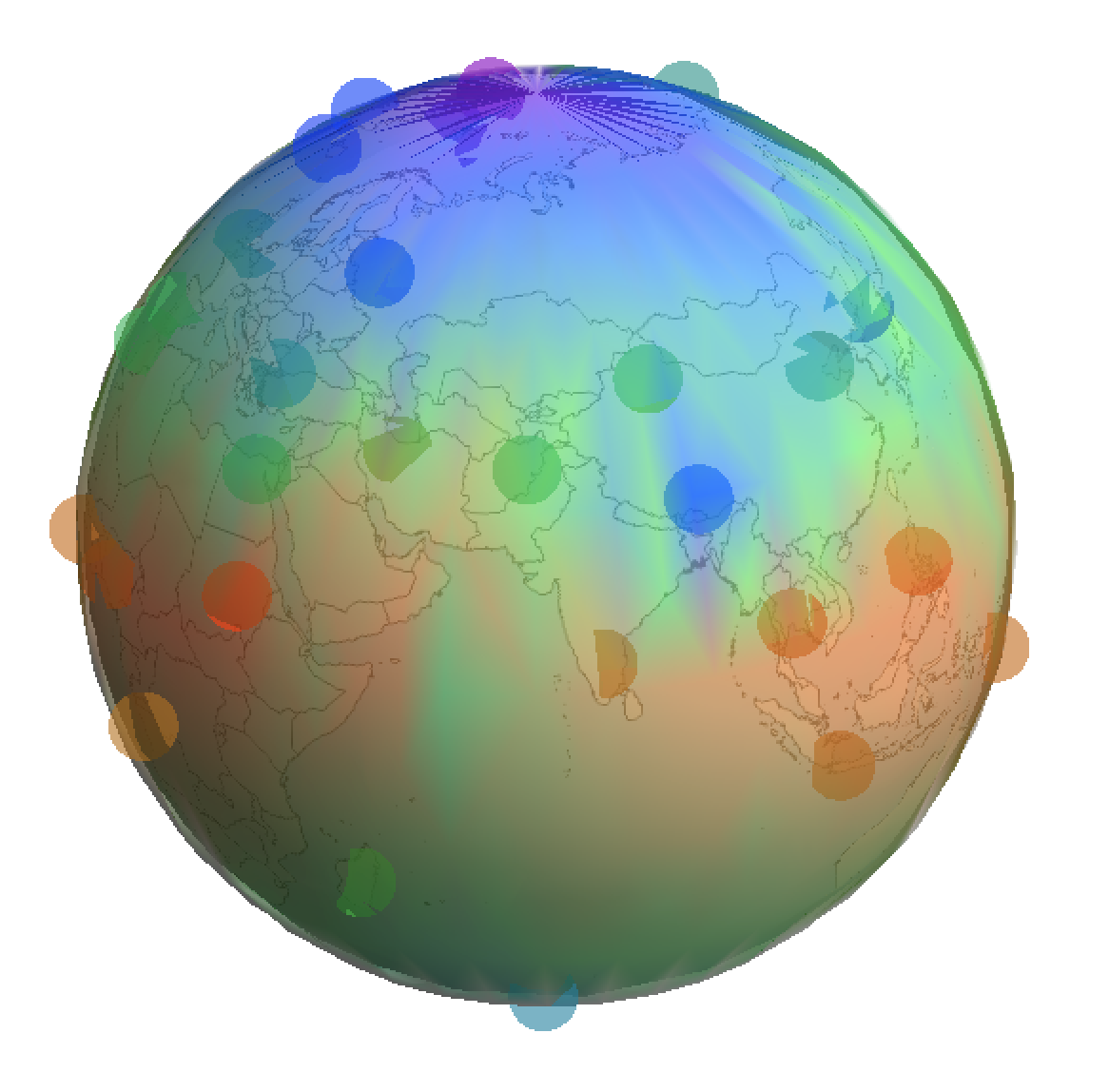### What?

Here's what this tournament looks like. Each point (other than the center) is an angle, and each arrow represents one angle beating another.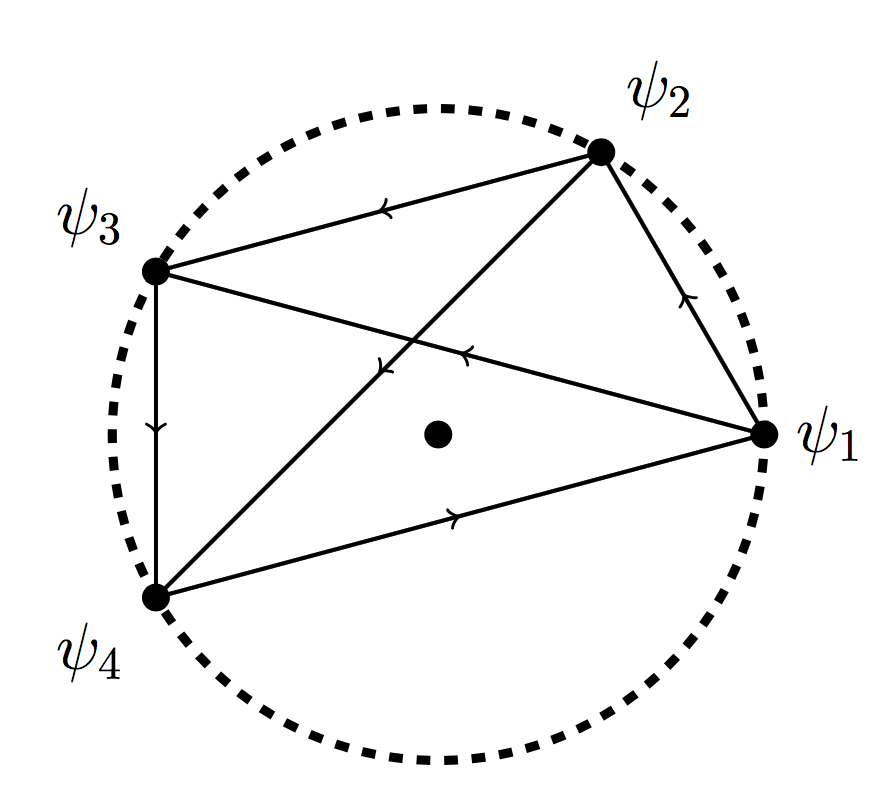My first claim above states that for any cycle, such as,, there is a shorter subcycle. In this case one such subcycle is.

My second claim says that since this tournament contains a cycle, there is no angle which beats every other angle. Each angle has at least one arrow pointing to it, so this holds true.

### First Claim: every cycle has a subcycle of length 3

Let's first argue that any cycle has a subcycle of length 3. Suppose the cyclehas length. If the cycle does multiple revolutions, then it must pass overat least once; it jumps from sometowhereis clockwise fromandis couterclockwise from it. Then we know thatis a shorter subcycle.

On the other hand, if the cycle does only one revolution, then consider each consecutive pair of angle shifts: the counterclockwise angle betweenand, betweenand, and so forth. Since the average single angle shift is, the average of these double shifts is. Since, one of these double angle shifts betweenandmust be at least. That means thatis counterclockwise from! Therefore we can simply skip, leaving us with a shorter cycle. This is illustrated in the example below, where the red edges can be replaced by the dotted edges.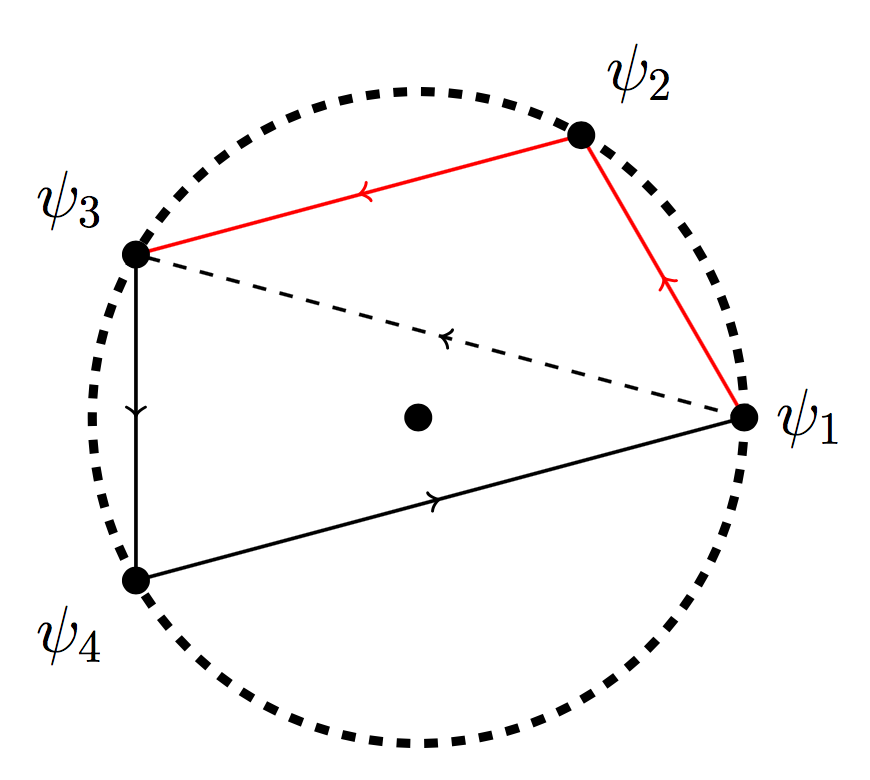Since any cycle of length greater than 3 can be shortened, we can continue shortening a cycle until we find its subcycle of length 3. That shows the first property.

### Second Claim: conditions when no cycle exists

We want to show that there is no cycle if and only if there exists an angle which every other angle beats, so consider the example below. Since every angle beats,beats nothing, and there is no cycle.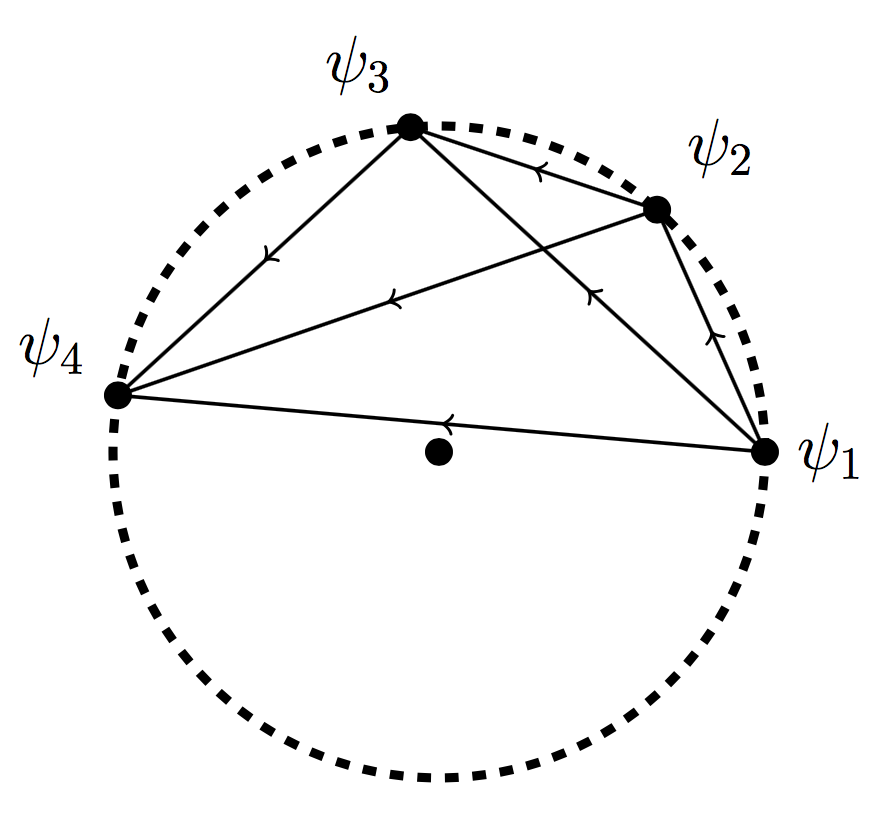If there is an anglewhich every other angle beats, then thatdoesn't beat anything. Then the entire swath of circle between anglesandmust be empty. Since an angle can only beat angles that are withinof it in the counterclockwise direction, no sequence of's can get past this swath, so there can be no cycle.

And if there is a cycle, then every angle on the cycle beats at least one other angle and is beaten by at least one other angle. Any angle not on the cycle must be between two points on the cycle, so must be less thanradians clockwise from one and less thanradians counterclockwise from the other. Therefore if a cycle exists, every angle has at least one edge going into it and one coming out. That shows the second property.

### Interpolation!

If you know the temperature at various weather stations around the globe and want to estimate it a some point, you will need to pick 3 surrounding weather stations to interpolate between. A human could (with a bit of difficulty) find a set of weather stations which encompass(or decide there is no such set), but how would you rigorously explain how you found those stations?

There are a few ways to program the solution. The first is Delaunay triangulation, in which we draw a bunch of non-intersecting lines between these weather stations so that the whole sphere is covered in spherical triangles. Then we simply choose the weather stations forming the vertices of the triangle surrounding our point. The advantage to this approach is that it gives you a consistent approximation every time you estimate the temperature at a new point on the globe. The disadvantage is that using Delaunay triangulation and determining which spherical trianglelies in are difficult programming challenges.

Another approach is to look directly at the point in question, then try to find a triangle surrounding it. Each weather station must be at some angle relative to this point, so the problem of finding such a triangle reduces to the problem of finding a cycle in one of the tournaments described above. We can determine whetherandgo in counterclockwise order by whether the determinant ofis negative, whereare the coordinates of weather stationsand. Using this for every pair of weather stations gives us a tournament, and our problem is to find a cycle in this tournament. Using the properties above, we can quickly deduce whether such a cycle exists, and some basic algorithms let us find a good cycle pretty quickly.

I implemented this second approach, taking temperature data from various cities on the globe today. I learned a bunch of capitals in the process.